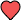# Resources

## How to Program the Mid-Point Formula on Your Graphing Calculator

The mid-point formula lets you find the point that is exactly midway between two other points. For instance, you might need to find a line that bisects (divides into two equal halves) a given line segment. This middle point is called the "midpoint".© 2019 Infinity Calcs | Made with